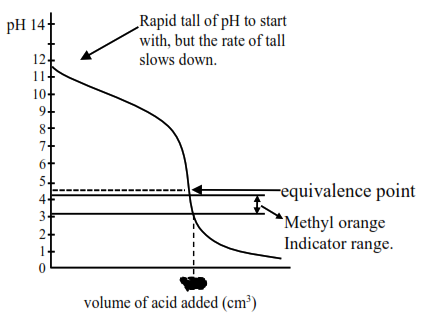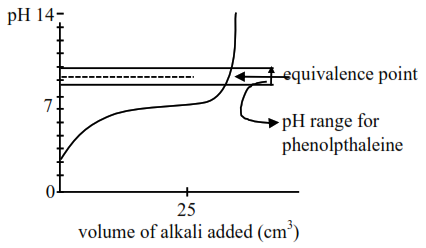# Given below are two statements.Question:

Given below are two statements.

Statement I: In the titration between strong acid and weak base methyl orange is suitable as an indicator.

Statement II: For titration of acetic acid with $\mathrm{NaOH}$ phenolphthalein is not a suitable indicator. In the light of the above statements, choose the most appropriate answer from the options given below:

1. Statement I is false but Statement II is true

2. Statement I is true but Statement II is false

3. Both Statement I and Statement II are true

4. Both Statement I and Statement II are false

Correct Option: , 2

Solution:

Titration curve for strong acid and weak base initially a buffer of weak base and conjugate acid is :Formed, thus $\mathrm{pH}$ falls slowly and after equivalence point, so the $\mathrm{pH}$ falls sharply so methyl arrange, having $\mathrm{pH}$ range of $3.2$ to $4.4$ will weak as indicator. So statement-I is correct.Titration curve for weak acid and strong base (NaOH)

Initially weak acid will form a buffer so $\mathrm{pH}$ increases slowly but after equivalence point. it rises sharply covering range of phenolphthalein so it will be suitable indicator so statement-II is false.